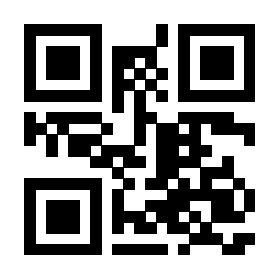# 正文与定制标题之间的间隔怎么缩小？\documentclass[11pt,a4paper,twoside,openright]{ctexbook}
%nummering
\usepackage{tikz}
\usetikzlibrary{matrix}
\usepackage{fancyhdr}
\pagestyle{fancy}
\pagestyle{plain}
\usepackage[dutch]{babel}
\usepackage[babel=true]{csquotes}
\usepackage[T1]{fontenc}
\usepackage[explicit]{titlesec}
\usepackage{xcolor}
\usepackage{charter}
\usepackage{amsmath, amsthm, amssymb, amsfonts, enumerate}

\definecolor{mybluei}{RGB}{5, 0, 100}
\definecolor{myblueii}{RGB}{0, 0, 100}
\usepackage{rotating, graphicx}
\usepackage{titlesec}
\usepackage{tcolorbox}
\newcommand\ChapterFont{\bfseries\rmfamily\selectfont\huge}
\newcommand\SectionFont{\bfseries\rmfamily\selectfont\Large}

\titleformat{\chapter}[block]
{\normalfont\ChapterFont\color{myblueii}}
{\tcbset{colframe=mybluei, boxrule=0.8pt, left=0pt, right=0pt, top=0pt, bottom=0pt}\hskip 0.25em\mbox{\tcbox[ boxsep=12pt, colback=mybluei, tcbox raise = -10pt]{\color{white}\bfseries\fontsize{65}{65}\selectfont\thechapter}}}
{0.5 em}
{#1\vskip0.6ex\endgraf\titlerule[1ex]}[]

\begin{document}

\chapter{测试测试测试}

\end{document}

0

227

\titleformat{\chapter}[block]
{\normalfont\ChapterFont\color{myblueii}}
{\tcbset{colframe=mybluei, boxrule=0.8pt, left=0pt, right=0pt, top=0pt, bottom=0pt}\hskip 0.25em\mbox{\tcbox[ boxsep=12pt, colback=mybluei, tcbox raise = -10pt]{\color{white}\bfseries\fontsize{65}{65}\selectfont\thechapter}}}
{0.5 em}
{#1\vskip0.6ex\endgraf\titlerule[1ex]}[\vskip-3em]
1 个回答

### 撰写答案